# Tips from an Online Physics Tutor: What is a Pascal? Understanding Pressure

A common problem for physics students is learning new measures and what they represent.  We use things like “seconds” to measure time in everyday life, so that isn’t too hard to conceptualize in physics class.  Likewise, though you may be more familiar with “pounds” than “kilograms,” or “feet” than “meters,” these measures of mass and distance are still fairly straightforward to talk about in class.  Other units, however, can be more difficult because they are new.  As a tutor and physics teacher, I found that if students have a strong understanding of new units and what they mean, it dramatically helps in both their physics understanding and their physics grades.

This is the third installment in discussions about new units in physics.  We have already learned about Newtons and the Joule.  Next on our list is the main unit of pressure called the Pascal.

Pascals measure pressure, which is a term that many students are already familiar with from a variety of contexts.  Usually, this knowledge is from things like tire pressure, or water pressure, or in situations like balloons or soda cans.  Our physics definition is that pressure is the perpendicular force on a surface over the area of the surface it is applied to.  We can see this in equation form: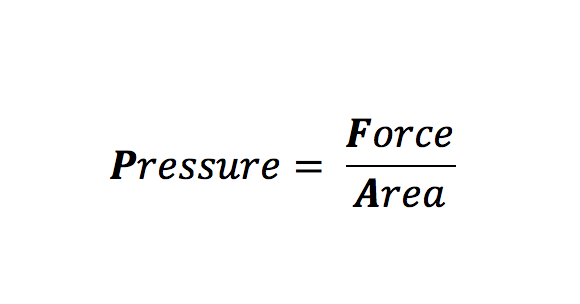In most of the common situations described above, the pressure is being created from the force of a gas pushing on the inside area of some container: air pushing on the inside of a tire or balloon, or strictly carbon dioxide in a soda can.  However, pressure does not only have to come from the air – notice that the equation on specifies a force and an area.  Think of filling a balloon with water instead of air, or even sand.  We get the same situation as the balloon expanding from the internal pressure, just a little differently because water and sand don’t expand in every direction like air.

Pressure can apply in a huge variety of situations.  Imagine pushing on a wall with your hand.  You likely won’t cause any damage to the wall by pushing on it because the force is spread out over the entire surface of your hand that is in contact with the wall – making a small pressure.  Now, imagine pushing with the same strength only this time with a nail or a needle.  You are much more likely to pierce the wall now, even though the same force was applied because now it is all given in a very small area.

While pressure is often related to gases, it is important to recognize that the fundamental components are just force and area.  Now, lets right this equation in terms of their units: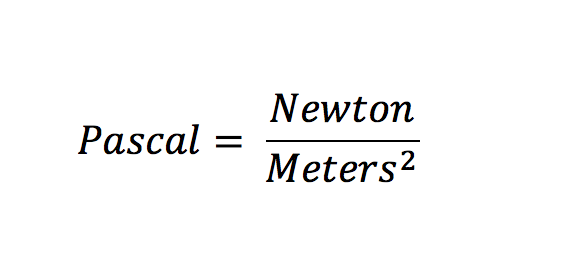Pascals are the units for pressure, newtons are the units for force, and square meters are the units for the area (think “square miles” or “square foot”).  So, we find that this pascal is really just a newton divided by meters squared.

This is a perfectly good way to think of a pascal, but remember that we can break it down into even more fundamental units if we remember that a newton can also be broken down into other units (**again, see here for the breakdown of the newton*****).  A newton is a kilogram times a meter, divided by seconds squared: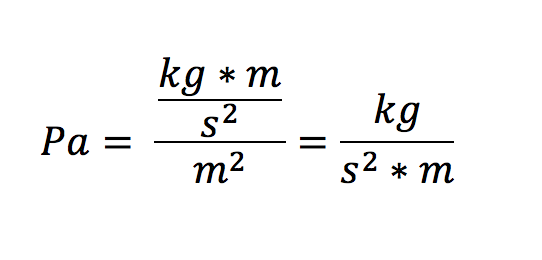So, a pascal can also be described as just kilograms per square seconds times meters.  This can be relevant for when we work with physics equations that incorporate pressure.  Here, we’ll look at two common physics equations: the ideal gas law, and the change in gravitational pressure.

First, the ideal gas law.  You may have already seen this in chemistry.  It describes the characteristics of ideal gases and how they relate to one another: PV =nRT.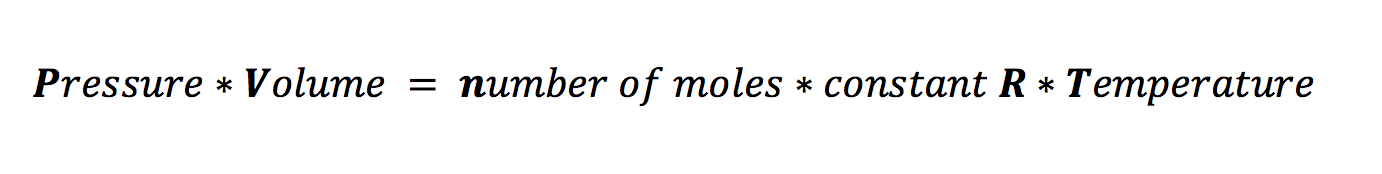To see how this equation works with our new definition of the pascal, we can just plug in the units for all of these variables and see if it makes sense.  First, pressures are in pascals – which we just found to be the volume is in cubic meters (m3), number of moles is in moles (mol), the constant R is 8.314-kilogram square meters per kelvin moles times square seconds (), and the physics unit for temperature is Kelvin (K).  This looks like a lot of units, especially because of the units on our constant term, but let’s see what happens when we plug it all in.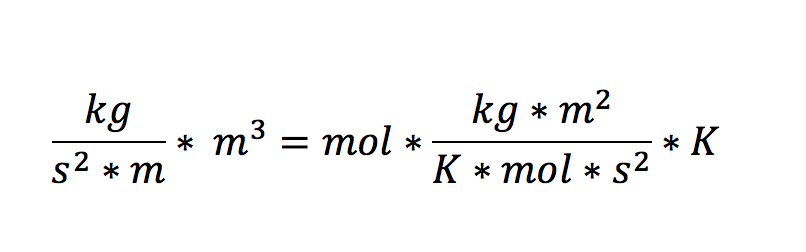Simplifying both sides gets us to: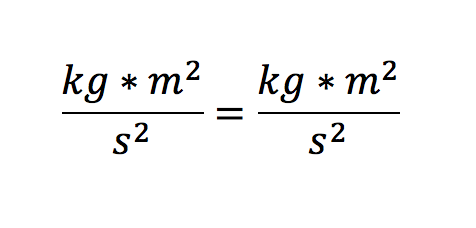One of the meters cancels out on the left, and the kelvin and moles cancel out on the right.  This leaves us with the exact same units on both sides, showing us how the ideal gas equation works.  This can also be helpful if you ever forget the units on the constant R (though note that it can be translates to different units depending on what you are using in class).

Next, we can look at the equation for gravitational pressure.  This tells you how much pressure you will feel from everything that is stacked above you. Typically, this is just the pressure from the air in the atmosphere, but it becomes much relevant when going underwater, or some other liquid.  This finds the change in pressure as you change in height in some substances like the air or ocean.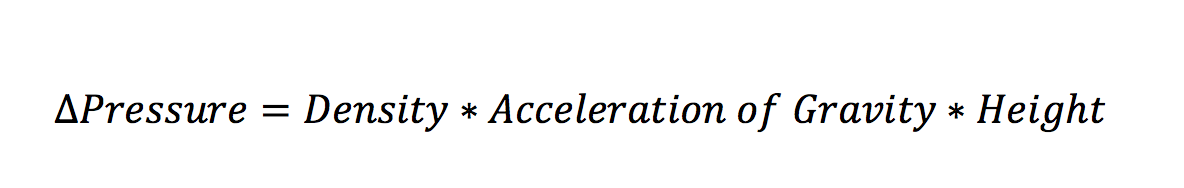This shows that the denser the material and the deeper you are in it, the greater change in pressure you will feel from the force of gravity pushing that mass down on you.  Let’s rewrite this as well in terms of their units.

The pressure is still kg/s^2*m; density is mass divided by volume (kg/m^3), the acceleration of gravity – little g – is 9.8 meters per second squared (m/s^2), and height is measured in meters (m).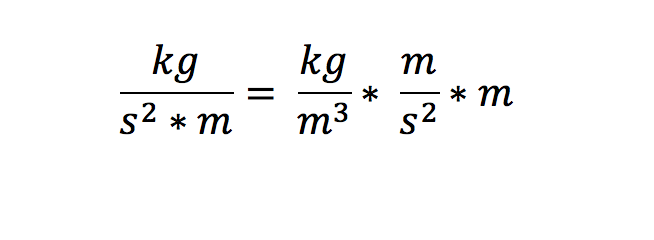When we simplify the right side, we get both meters on the top of the fraction bar canceling out, leaving us just with: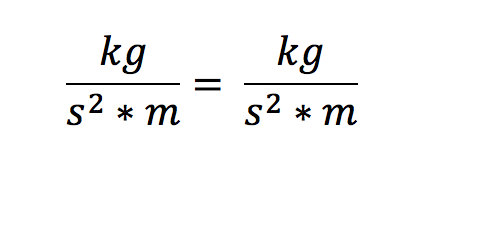This, of course, is our same equation for pressure.  So, we’ve found the fundamental units of pressure with three very different equations and observed how the units work.  Thinking of pressure as a force over an area (or newtons per square meter) is likely a simpler conceptual definition, and thinking of it as kilograms per meter squared second can be beneficial in checking and understanding equations.

Learning how new units like the pascal can be broken down into fundamental units can help with your understanding of physics and your execution of equations when taking tests or solving problems.  Taking a little time to break up new and confusing terms into their derivations is always a good practice that will leave you with a deeper and more memorable understanding of the topic. Knowing all of your measures and units alone can be a huge benefit in early physics classes.

Michael C. is currently a private online math, science, and standardized test tutor with TutorNerd in Irvine and Anaheim.